Mivoc

Mivoc has chassis with a very good price/performance ratio. They come from the company Speaker-Trade.

They are no longer available for DIY.

TweeterWooferopen a bigger photo

recommended cabinet 1:
closed cabinet with 0,86 L volume
from 129/81 Hz (-3dB/-8dB)

recommended cabinet 2:
2,6 L volume bass reflex cabinet
with HP35 reflex tube, 21 cm long.
from 70/54 Hz (-3dB/-8dB).

Mivoc XAW 110 HC-II Black

order no. rm-xaw110hc-black

EUR 45,20excl. VAT: € 37.98 / \$ 43.30

11 cm midrange driver with high quality cone.

• power handling (continuous/programme) = 60/150 W
• frequency range = 40-3500 Hz
• resonance frequency fs = 50 Hz
• impedance R = 8 Ohm
• sound pressure level SPL = 86 dB (2,83V; 1m)
• DC resistance Re = 6,2 Ohm
• force factor BL = 6,5 N/A
• effective piston radiating area Sd = 54 cm2
• effective mechanical mass incl. air load mms = 7,2 g
• equivalent volume of compliance Vas = 5,8 l
• total Q factor Qts = 0,31 (Qms=5, Qes=0,33)
• voice coil diameter = 25 mm
• maximum peak linear excursion vibration xlin = +/- 3 mm
• mounting diameter d = 92 mm
• overall diameter d = 124 mm
• mounting depth (not countersunk) t = 56 mm
PDF data sheet

Subwooferrecommended cabinet 1:
closed cabinet with 8,1 L volume
from 74/47 Hz (-3dB/-8dB)

recommended cabinet 2:
29 L volume bass reflex cabinet
with HP70 reflex tube, 20 cm long.
from 37/30 Hz (-3dB/-8dB).

Mivoc XAW 180 HC black

order no. rm-xaw180hc-black

EUR 48,20excl. VAT: € 40.50 / \$ 46.17

17 cm bass driver with high quality cone. The basket is made of die-cast.

• power handling (continuous/programme) = 80/200 W
• frequency range = 40-3000 Hz
• resonance frequency fs = 35 Hz
• impedance R = 8 Ohm
• sound pressure level SPL = 87,5 dB (2,83V; 1m)
• DC resistance Re = 5,6 Ohm
• force factor BL = 6,7 N/A
• voice coil inductance L = 0,5 mH
• effective piston radiating area Sd = 132 cm2
• effective mechanical mass incl. air load mms = 15,1 g
• equivalent volume of compliance Vas = 33,5 l
• total Q factor Qts = 0,38 (Qms=4,5, Qes=0,41)
• voice coil diameter = 25 mm
• maximum peak linear excursion vibration xlin = +/- 4,2 mm
• mounting diameter d = 145 mm
• overall diameter d = 187 mm
• mounting depth (not countersunk) t = 98 mm
PDF data sheetopen a bigger photo

recommended cabinet 1:
closed cabinet with 8,4 L volume
from 85/53 Hz (-3dB/-8dB)

recommended cabinet 2:
30 L volume bass reflex cabinet
with HP70 reflex tube, 14 cm long.
from 43/34 Hz (-3dB/-8dB).

Mivoc XAW 208 HC

order no. rm-xaw208hc- not available

21 cm bass driver with high quality cone. The basket is made of die-cast.

• power handling (continuous/programme) = 120/300 W
• frequency range = 38-2000 Hz
• resonance frequency fs = 40 Hz
• impedance R = 8 Ohm
• sound pressure level SPL = 89,5 dB (2,83V; 1m)
• DC resistance Re = 6,1 Ohm
• force factor BL = 9,8 N/A
• voice coil inductance L = 0,6 mH
• effective piston radiating area Sd = 201 cm2
• effective mechanical mass incl. air load mms = 25,8 g
• equivalent volume of compliance Vas = 34,7 l
• total Q factor Qts = 0,38 (Qms=5,1, Qes=0,41)
• voice coil diameter = 37 mm
• maximum peak linear excursion vibration xlin = +/- 4,5 mm
• mounting diameter d = 183 mm
• overall diameter d = 225 mm
• mounting depth (not countersunk) t = 105 mm
PDF data sheet

no longer soldopen a bigger photo

recommended cabinet 1:
closed cabinet with 3,4 L volume
from 92/58 Hz (-3dB/-8dB)

recommended cabinet 2:
12,8 L volume bass reflex cabinet
with HP50 reflex tube, 16 cm long.
from 45/37 Hz (-3dB/-8dB).

Mivoc SWM68

order no. not-available- not available

15 cm bass driver with paper cone.

• power handling (continuous/programme) = 120/200 W
• frequency range = 35-1500 Hz
• resonance frequency fs = 47 Hz
• impedance R = 8 Ohm
• sound pressure level SPL = 86 dB (2,83V; 1m)
• DC resistance Re = 6,6 Ohm
• force factor BL = 9 N/A
• voice coil inductance L = 0,65 mH
• effective piston radiating area Sd = 124,7 cm2
• effective mechanical mass incl. air load mms = 21,7 g
• equivalent volume of compliance Vas = 11,5 l
• total Q factor Qts = 0,41 (Qms=2, Qes=0,52)
• voice coil diameter = 38 mm
• maximum peak linear excursion vibration xlin = +/- 6,3 mm
• mounting diameter d = 132 mm
• overall diameter d = 160 mm
• mounting depth (not countersunk) t = 78 mm
PDF data sheetMivoc KFT 130 M

order no. not-available- not available

ribbon tweeter. The magnet system is based on Neodymium technology. The driver is magnetically shielded.

• power handling (continuous/programme) = 20 W
• frequency range = 1200-40000 Hz
• resonance frequency fs = 1100 Hz
• impedance R = 8 Ohm
• sound pressure level SPL = 93 dB (2,83V; 1m)
• DC resistance Re = 7 Ohm
• effective piston radiating area Sd = 12,5 cm2
• overall diameter d = 135x98 mm
• mounting depth (not countersunk) t = 40 mm
PDF data sheetMivoc HGH 258 FN-II

order no. not-available- not available

25 mm dome tweeter with silk dome. The voice coil windings are immersed in magnetic fluid to increase short term power handling capacity, to reduce the compression and the Q factor..

• power handling (continuous/programme) = 120/300@>2,5kHz/12dB W
• frequency range = 2000-22000 Hz
• resonance frequency fs = 1500 Hz
• impedance R = 8 Ohm
• sound pressure level SPL = 93 dB (2,83V; 1m)
• DC resistance Re = 6,6 Ohm
• effective piston radiating area Sd = 4,9 cm2
• voice coil diameter = 25 mm
• mounting diameter d = 49 mm
• overall diameter d = 70 mm
• mounting depth (not countersunk) t = 39 mm
PDF data sheetMivoc HGH 258 FN-II Titan

order no. not-available- not available

25 mm dome tweeter with silk dome. The voice coil windings are immersed in magnetic fluid to increase short term power handling capacity, to reduce the compression and the Q factor..

• power handling (continuous/programme) = 120/300@>2,5kHz/12dB W
• frequency range = 2000-22000 Hz
• resonance frequency fs = 1500 Hz
• impedance R = 8 Ohm
• sound pressure level SPL = 93 dB (2,83V; 1m)
• DC resistance Re = 6,6 Ohm
• effective piston radiating area Sd = 4,9 cm2
• voice coil diameter = 25 mm

Mivoc FR358N

order no. not-available- not available

full range driver with paper cone. The basket is made of die-cast. The magnet system is based on Neodymium technology.

• power handling (continuous/programme) = 50/80 W
• frequency range = 80-20000 Hz
• resonance frequency fs = 88 Hz
• impedance R = 8 Ohm
• sound pressure level SPL = 89 dB (2,83V; 1m)
• DC resistance Re = 7,3 Ohm
• force factor BL = 5,2 N/A
• voice coil inductance L = 0,23 mH
• effective piston radiating area Sd = 36,3 cm2
• effective mechanical mass incl. air load mms = 2,4 g
• equivalent volume of compliance Vas = 2,5 l
• total Q factor Qts = 0,32 (Qms=3,2, Qes=0,36)
• voice coil diameter = 25 mm
• maximum peak linear excursion vibration xlin = +/- 3 mm
• mounting diameter d = 77 mm
• overall diameter d = 86x86 mm
• mounting depth (not countersunk) t = 51 mm
PDF data sheetMivoc HPA248

order no. not-available- not available

25 mm driver with high quality cone.

• power handling (continuous/programme) = 20012dB/4kHz W
• frequency range = 2800-20000 Hz
• resonance frequency fs = 1900 Hz
• impedance R = 8 Ohm
• sound pressure level SPL = 106 dB (2,83V; 1m)
• DC resistance Re = 6,5 Ohm
• voice coil diameter = 25 mm
• mounting diameter d = 170x94 mm
• overall diameter d = 192x114 mm
• mounting depth (not countersunk) t = 11,3 mm
PDF data sheet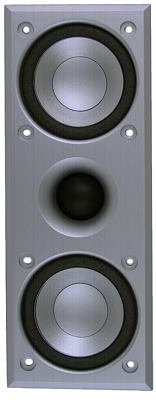Mivoc MHE280 silver

order no. not-available- not available

.

• frequency range = 60-22000 Hz
• impedance R = 8 Ohm
• sound pressure level SPL = 89 dB (2,83V; 1m)
• overall diameter d = 211x81 mm
• mounting depth (not countersunk) t = 41 mmMivoc MHE280 black

order no. not-available- not available

.

• frequency range = 60-22000 Hz
• impedance R = 8 Ohm
• sound pressure level SPL = 89 dB (2,83V; 1m)
• overall diameter d = 211x81 mm
• mounting depth (not countersunk) t = 41 mmopen a bigger photo

recommended cabinet 1:
closed cabinet with 3,1 L volume
from 88/56 Hz (-3dB/-8dB)

recommended cabinet 2:
12,3 L volume bass reflex cabinet
with HP50 reflex tube, 18 cm long.
from 40/34 Hz (-3dB/-8dB).

Mivoc WAL 416

order no. not-available- not available

.

• power handling (continuous/programme) = 60/150 W
• frequency range = 50-7000 Hz
• resonance frequency fs = 61 Hz
• impedance R = 16 Ohm
• sound pressure level SPL = 83 dB (2,83V; 1m)
• DC resistance Re = 11,2 Ohm
• force factor BL = 6,9 N/A
• effective piston radiating area Sd = 53 cm2
• effective mechanical mass incl. air load mms = 6,9 g
• equivalent volume of compliance Vas = 4 l
• total Q factor Qts = 0,56 (Qms=5,45, Qes=0,62)
• voice coil diameter = 25 mm
• maximum peak linear excursion vibration xlin = +/- 5 mm
• mounting diameter d = 94 mm
• overall diameter d = 116 mm
• mounting depth (not countersunk) t = 63 mm
PDF data sheetrecommended cabinet 1:
closed cabinet with 4,5 L volume
from 93/59 Hz (-3dB/-8dB)

recommended cabinet 2:
18 L volume bass reflex cabinet
with HP50 reflex tube, 10 cm long.
from 45/37 Hz (-3dB/-8dB).

Mivoc WPT 138

order no. not-available- not available

13 cm midrange driver with polypropylene cone.

• power handling (continuous/programme) = 30/80 W
• frequency range = 60-3000 Hz
• resonance frequency fs = 52 Hz
• impedance R = 8 Ohm
• sound pressure level SPL = 83 dB (2,83V; 1m)
• DC resistance Re = 6,5 Ohm
• force factor BL = 5,6 N/A
• voice coil inductance L = 0,53 mH
• effective piston radiating area Sd = 82 cm2
• effective mechanical mass incl. air load mms = 7,5 g
• equivalent volume of compliance Vas = 12 l
• total Q factor Qts = 0,45 (Qms=3,7, Qes=0,51)
• voice coil diameter = 25 mm
• maximum peak linear excursion vibration xlin = +/- 3,5 mm
• mounting diameter d = 112 mm
• overall diameter d = 145 mm
• mounting depth (not countersunk) t = 62 mm
PDF data sheetrecommended cabinet 1:
closed cabinet with 5,5 L volume
from 118/75 Hz (-3dB/-8dB)

recommended cabinet 2:
16 L volume bass reflex cabinet
with HP70 reflex tube, 14 cm long.
from 66/51 Hz (-3dB/-8dB).

Mivoc mpa WM8

order no. not-available- not available

20 cm bass driver with high quality cone.

• power handling (continuous/programme) = 120/300 W
• frequency range = 60-3000 Hz
• resonance frequency fs = 43 Hz
• impedance R = 8 Ohm
• sound pressure level SPL = 92 dB (2,83V; 1m)
• DC resistance Re = 6,6 Ohm
• force factor BL = 11 N/A
• voice coil inductance L = 0,2 mH
• effective piston radiating area Sd = 214 cm2
• effective mechanical mass incl. air load mms = 20,4 g
• equivalent volume of compliance Vas = 43 l
• total Q factor Qts = 0,29 (Qms=6,1, Qes=0,3)
• voice coil diameter = 38 mm
• maximum peak linear excursion vibration xlin = +/- 5 mm
• mounting diameter d = 183 mm
• overall diameter d = 220 mm
• mounting depth (not countersunk) t = 97 mm
PDF data sheet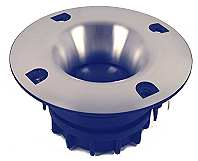Mivoc XGH 258 Alu

order no. not-available- not available

25 mm dome tweeter with silk dome. The voice coil windings are immersed in magnetic fluid to increase short term power handling capacity, to reduce the compression and the Q factor..

• power handling (continuous/programme) = 120/300@>2,5kHz/12dB W
• frequency range = 2000-22000 Hz
• resonance frequency fs = 1300 Hz
• impedance R = 8 Ohm
• sound pressure level SPL = 93 dB (2,83V; 1m)
• DC resistance Re = 6,6 Ohm
• effective piston radiating area Sd = 4,9 cm2
• voice coil diameter = 25 mm
• mounting diameter d = 50 mm
• overall diameter d = 75 mm
• mounting depth (not countersunk) t = 41 mm
PDF data sheet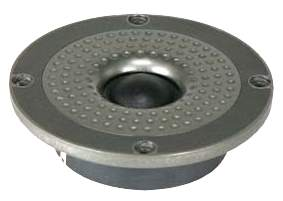Mivoc HG 258 G, Titan

order no. not-available- not available

25 mm dome tweeter with silk dome. The voice coil windings are immersed in magnetic fluid to increase short term power handling capacity, to reduce the compression and the Q factor..

• power handling (continuous/programme) = 120/300@>2,5kHz/12dB W
• frequency range = 2000-22000 Hz
• resonance frequency fs = 1600 Hz
• impedance R = 8 Ohm
• sound pressure level SPL = 91 dB (2,83V; 1m)
• DC resistance Re = 7 Ohm
• effective piston radiating area Sd = 4,9 cm2
• voice coil diameter = 25 mm
• mounting diameter d = 70 mm
• overall diameter d = 104 mm
• mounting depth (not countersunk) t = 31 mm
PDF data sheetMivoc HG 258 G, Schwarz

order no. not-available- not available

25 mm dome tweeter with silk dome. The voice coil windings are immersed in magnetic fluid to increase short term power handling capacity, to reduce the compression and the Q factor..

• power handling (continuous/programme) = 120/300@>2,5kHz/12dB W
• frequency range = 2000-22000 Hz
• resonance frequency fs = 1600 Hz
• impedance R = 8 Ohm
• sound pressure level SPL = 91 dB (2,83V; 1m)
• DC resistance Re = 7 Ohm
• effective piston radiating area Sd = 4,9 cm2
• voice coil diameter = 25 mm
• mounting diameter d = 70 mm
• overall diameter d = 104 mm
• mounting depth (not countersunk) t = 31 mm
PDF data sheetMivoc HPA268 neo

order no. not-available- not available

25 mm driver with high quality cone.

• power handling (continuous/programme) = 20012dB/3,5kHz W
• frequency range = 2500-20000 Hz
• resonance frequency fs = 1800 Hz
• impedance R = 8 Ohm
• sound pressure level SPL = 104 dB (2,83V; 1m)
• DC resistance Re = 6,5 Ohm
• voice coil diameter = 25 mm
• mounting diameter d = 150x112 mm
• overall diameter d = 196x156 mm
• mounting depth (not countersunk) t = 12,3 mm
PDF data sheet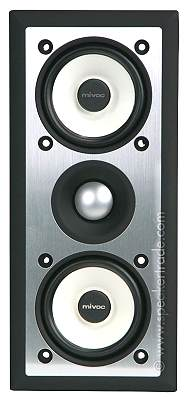Mivoc MHE380 Alu

order no. not-available- not available

.

• frequency range = 60-23000 Hz
• resonance frequency fs = 91 Hz
• impedance R = 8 Ohm
• sound pressure level SPL = 91 dB (2,83V; 1m)
• force factor BL = 3,5 N/A
• effective mechanical mass incl. air load mms = 1,75 g
• equivalent volume of compliance Vas = 1,1 l
• total Q factor Qts = 0,48 (Qms=6,6, Qes=0,52)
• voice coil diameter = 19 mm
• maximum peak linear excursion vibration xlin = +/- 1,5 mm
• overall diameter d = 226x100 mm
• mounting depth (not countersunk) t = 77 mm
PDF data sheetMivoc MHE380 black

order no. not-available- not available

.

• frequency range = 60-23000 Hz
• resonance frequency fs = 91 Hz
• impedance R = 8 Ohm
• sound pressure level SPL = 91 dB (2,83V; 1m)
• force factor BL = 3,5 N/A
• effective mechanical mass incl. air load mms = 1,75 g
• equivalent volume of compliance Vas = 1,1 l
• total Q factor Qts = 0,48 (Qms=6,6, Qes=0,52)
• voice coil diameter = 19 mm
• maximum peak linear excursion vibration xlin = +/- 1,5 mm
• overall diameter d = 226x100 mm
• mounting depth (not countersunk) t = 77 mm
PDF data sheetMivoc WPN 80

order no. not-available- not available

7 cm midrange driver with paper cone.

• power handling (continuous/programme) = 30/80 W
• frequency range = 88-12000 Hz
• resonance frequency fs = 88 Hz
• impedance R = 8 Ohm
• sound pressure level SPL = 83 dB (2,83V; 1m)
• DC resistance Re = 6,2 Ohm
• force factor BL = 2,8 N/A
• voice coil inductance L = 0,2 mH
• effective piston radiating area Sd = 23,8 cm2
• effective mechanical mass incl. air load mms = 2 g
• equivalent volume of compliance Vas = 1,3 l
• total Q factor Qts = 0,71 (Qms=3,73, Qes=0,88)
• voice coil diameter = 20 mm
• maximum peak linear excursion vibration xlin = +/- 1,76 mm
• mounting diameter d = 60 mm
• overall diameter d = 70 x 70 mm
• mounting depth (not countersunk) t = 35 mmrecommended cabinet 1:
closed cabinet with 0,9 L volume
from 127/80 Hz (-3dB/-8dB)

recommended cabinet 2:
3 L volume bass reflex cabinet
with HP35 reflex tube, 19 cm long.
from 67/52 Hz (-3dB/-8dB).

Mivoc XAW 110 HC

order no. not-available- not available

11 cm midrange driver with high quality cone.

• power handling (continuous/programme) = 60/150 W
• frequency range = 40-3500 Hz
• resonance frequency fs = 54 Hz
• impedance R = 8 Ohm
• sound pressure level SPL = 86 dB (2,83V; 1m)
• DC resistance Re = 6 Ohm
• force factor BL = 6,4 N/A
• voice coil inductance L = 0,4 mH
• effective piston radiating area Sd = 54 cm2
• effective mechanical mass incl. air load mms = 7,3 g
• equivalent volume of compliance Vas = 4,9 l
• total Q factor Qts = 0,34 (Qms=4,9, Qes=0,36)
• voice coil diameter = 25 mm
• maximum peak linear excursion vibration xlin = +/- 3 mm
• mounting diameter d = 92 mm
• overall diameter d = 124 mm
• mounting depth (not countersunk) t = 77 mm
PDF data sheetrecommended cabinet 1:
closed cabinet with 3,2 L volume
from 96/61 Hz (-3dB/-8dB)

recommended cabinet 2:
11,8 L volume bass reflex cabinet
with HP50 reflex tube, 15 cm long.
from 48/39 Hz (-3dB/-8dB).

Mivoc WPP 150

order no. not-available- not available

13 cm bass driver with polypropylene cone. The cone is equipped with a phase plug. The driver is magnetically shielded.

• power handling (continuous/programme) = 70/160 W
• resonance frequency fs = 48 Hz
• impedance R = 8 Ohm
• DC resistance Re = 6 Ohm
• force factor BL = 5,4 N/A
• voice coil inductance L = 0,5 mH
• effective piston radiating area Sd = 77 cm2
• effective mechanical mass incl. air load mms = 8 g
• equivalent volume of compliance Vas = 11,5 l
• total Q factor Qts = 0,4 (Qms=2,1, Qes=0,49)
• maximum peak linear excursion vibration xlin = +/- 3,4 mm
• mounting diameter d = 112 mm
• overall diameter d = 150 mm
• mounting depth (not countersunk) t = 76 mm
PDF data sheetrecommended cabinet 1:
closed cabinet with 6,2 L volume
from 90/57 Hz (-3dB/-8dB)

recommended cabinet 2:
24 L volume bass reflex cabinet
with HP70 reflex tube, 16 cm long.
from 45/36 Hz (-3dB/-8dB).

Mivoc WPP 180

order no. not-available- not available

16 cm bass driver with polypropylene cone. The cone is equipped with a phase plug.

• power handling (continuous/programme) = 80/200 W
• resonance frequency fs = 46 Hz
• impedance R = 8 Ohm
• DC resistance Re = 6 Ohm
• force factor BL = 6,95 N/A
• voice coil inductance L = 0,5 mH
• effective piston radiating area Sd = 130 cm2
• effective mechanical mass incl. air load mms = 13,1 g
• equivalent volume of compliance Vas = 21,2 l
• total Q factor Qts = 0,41 (Qms=1,9, Qes=0,52)
• maximum peak linear excursion vibration xlin = +/- 3,8 mm
• mounting diameter d = 142 mm
• overall diameter d = 180 mm
• mounting depth (not countersunk) t = 92 mm
PDF data sheetrecommended cabinet 1:
closed cabinet with 8,1 L volume
from 74/47 Hz (-3dB/-8dB)

recommended cabinet 2:
29 L volume bass reflex cabinet
with HP70 reflex tube, 20 cm long.
from 37/30 Hz (-3dB/-8dB).

Mivoc XAW 180 HC

order no. not-available- not available

17 cm bass driver with high quality cone. The basket is made of die-cast.

• power handling (continuous/programme) = 80/200 W
• frequency range = 40-3000 Hz
• resonance frequency fs = 35 Hz
• impedance R = 8 Ohm
• sound pressure level SPL = 87,5 dB (2,83V; 1m)
• DC resistance Re = 5,6 Ohm
• force factor BL = 6,7 N/A
• voice coil inductance L = 0,5 mH
• effective piston radiating area Sd = 132 cm2
• effective mechanical mass incl. air load mms = 15,1 g
• equivalent volume of compliance Vas = 33,5 l
• total Q factor Qts = 0,38 (Qms=4,5, Qes=0,41)
• voice coil diameter = 25 mm
• maximum peak linear excursion vibration xlin = +/- 4,2 mm
• mounting diameter d = 145 mm
• overall diameter d = 187 mm
• mounting depth (not countersunk) t = 98 mm
PDF data sheetrecommended cabinet 1:
closed cabinet with 3,6 L volume
from 94/59 Hz (-3dB/-8dB)

recommended cabinet 2:
9,5 L volume bass reflex cabinet
with HP70 reflex tube, 44 cm long.
from 54/41 Hz (-3dB/-8dB).

Mivoc XAW 210 HC

order no. not-available- not available

21 cm bass driver with high quality cone. The basket is made of die-cast.

• power handling (continuous/programme) = 120/300 W
• frequency range = 40-1000 Hz
• resonance frequency fs = 32 Hz
• impedance R = 4 Ohm
• sound pressure level SPL = 87,1 dB (2,83V; 1m)
• DC resistance Re = 3 Ohm
• force factor BL = 9,9 N/A
• voice coil inductance L = 1,3 mH
• effective piston radiating area Sd = 209 cm2
• effective mechanical mass incl. air load mms = 45,8 g
• equivalent volume of compliance Vas = 33 l
• total Q factor Qts = 0,27 (Qms=5,3, Qes=0,28)
• voice coil diameter = 37 mm
• maximum peak linear excursion vibration xlin = +/- 7,75 mm
• mounting diameter d = 185 mm
• overall diameter d = 225 mm
• mounting depth (not countersunk) t = 98 mm
PDF data sheet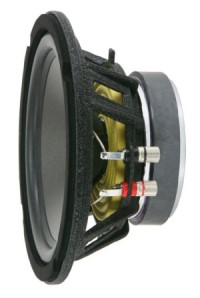Mivoc AW 2000

order no. not-available- not available

20 cm bass driver with high quality cone. The basket is made of die-cast.

• power handling (continuous/programme) = 120/300 W
• frequency range = 40-500 Hz
• resonance frequency fs = 30 Hz
• impedance R = 4 Ohm
• sound pressure level SPL = 88 dB (2,83V; 1m)
• DC resistance Re = 3 Ohm
• force factor BL = 9,3 N/A
• voice coil inductance L = 1,1 mH
• effective piston radiating area Sd = 214 cm2
• effective mechanical mass incl. air load mms = 49 g
• equivalent volume of compliance Vas = 37 l
• total Q factor Qts = 0,3 (Qms=4,1, Qes=0,32)
• voice coil diameter = 38 mm
• maximum peak linear excursion vibration xlin = +/- 6,5 mm
PDF data sheetrecommended cabinet 1:
closed cabinet with 4,6 L volume
from 80/51 Hz (-3dB/-8dB)

recommended cabinet 2:
16 L volume bass reflex cabinet
with HP70 reflex tube, 35 cm long.
from 41/33 Hz (-3dB/-8dB).

Mivoc AWM 104

order no. not-available- not available

25 cm bass driver with high quality cone. The basket is made of die-cast.

• power handling (continuous/programme) = 200/500 W
• frequency range = 50-300 Hz
• resonance frequency fs = 36 Hz
• impedance R = 4 Ohm
• sound pressure level SPL = 88 dB (2,83V; 1m)
• DC resistance Re = 3 Ohm
• force factor BL = 16,3 N/A
• voice coil inductance L = 2,3 mH
• effective piston radiating area Sd = 346 cm2
• effective mechanical mass incl. air load mms = 119 g
• equivalent volume of compliance Vas = 21,8 l
• total Q factor Qts = 0,34 (Qms=4,65, Qes=0,37)
• voice coil diameter = 50 mm
• maximum peak linear excursion vibration xlin = +/- 9 mm
• mounting diameter d = 234 mm
• overall diameter d = 270 mm
• mounting depth (not countersunk) t = 158 mm
PDF data sheetrecommended cabinet 1:
closed cabinet with 12,9 L volume
from 75/47 Hz (-3dB/-8dB)

recommended cabinet 2:
40 L volume bass reflex cabinet
with HP100 reflex tube, 33 cm long.
from 40/31 Hz (-3dB/-8dB).

Mivoc XAW 310 HC

order no. not-available- not available

31 cm bass driver with high quality cone. The basket is made of die-cast.

• power handling (continuous/programme) = 225/600 W
• frequency range = 30-500 Hz
• resonance frequency fs = 30 Hz
• impedance R = 4 Ohm
• sound pressure level SPL = 90 dB (2,83V; 1m)
• DC resistance Re = 2,8 Ohm
• force factor BL = 13,7 N/A
• voice coil inductance L = 1,9 mH
• effective piston radiating area Sd = 489 cm2
• effective mechanical mass incl. air load mms = 116 g
• equivalent volume of compliance Vas = 81 l
• total Q factor Qts = 0,31 (Qms=4,9, Qes=0,33)
• voice coil diameter = 63 mm
• maximum peak linear excursion vibration xlin = +/- 10 mm
• mounting diameter d = 282 mm
• overall diameter d = 336 mm
• mounting depth (not countersunk) t = 173 mm
PDF data sheetopen a bigger photo

recommended cabinet 1:
closed cabinet with 27 L volume
from 47/29 Hz (-3dB/-8dB)

recommended cabinet 2:
100 L volume bass reflex cabinet
with HP100 reflex tube, 34 cm long.
from 23/19 Hz (-3dB/-8dB).

Mivoc XAW 320 HC

order no. not-available- not available

30 cm bass driver with high quality cone. The basket is made of die-cast.

• power handling (continuous/programme) = 220/600 W
• frequency range = fs-300 Hz
• resonance frequency fs = 22,6 Hz
• impedance R = 4 Ohm
• sound pressure level SPL = 90 dB (2,83V; 1m)
• DC resistance Re = 3,2 Ohm
• force factor BL = 13,7 N/A
• voice coil inductance L = 0,8 mH
• effective piston radiating area Sd = 499 cm2
• effective mechanical mass incl. air load mms = 163 g
• equivalent volume of compliance Vas = 106 l
• total Q factor Qts = 0,37 (Qms=4,2, Qes=0,41)
• voice coil diameter = 63 mm
• maximum peak linear excursion vibration xlin = +/- 11 mm
• mounting diameter d = 284 mm
• overall diameter d = 312 mm
• mounting depth (not countersunk) t = 165 mm
PDF data sheet

Mivoc XAW 320 PR

order no. not-available- not availablerecommended cabinet 1:
closed cabinet with 22 L volume
from 61/39 Hz (-3dB/-8dB)

recommended cabinet 2:
81 L volume bass reflex cabinet
with HP100 reflex tube, 22 cm long.
from 31/25 Hz (-3dB/-8dB).

Raveland TW 3000

order no. not-available- not available

30 cm bass driver with high quality cone.

• power handling (continuous/programme) = 180/450 W
• resonance frequency fs = 29 Hz
• impedance R = 4 Ohm
• sound pressure level SPL = 92 dB (2,83V; 1m)
• DC resistance Re = 2,8 Ohm
• effective piston radiating area Sd = 499 cm2
• equivalent volume of compliance Vas = 93 l
• total Q factor Qts = 0,36 (Qms=3,8, Qes=0,4)
• maximum peak linear excursion vibration xlin = +/- 6 mm
• mounting diameter d = 284 mm
• overall diameter d = 312 mm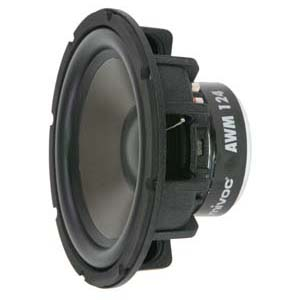recommended cabinet 1:
closed cabinet with 5,3 L volume
from 96/61 Hz (-3dB/-8dB)

recommended cabinet 2:
15 L volume bass reflex cabinet
with HP100 reflex tube, 58 cm long.
from 54/41 Hz (-3dB/-8dB).

Mivoc AWM 124

order no. not-available- not available

30 cm bass driver with high quality cone. The basket is made of die-cast.

• power handling (continuous/programme) = 220/600 W
• frequency range = 40-300 Hz
• resonance frequency fs = 35 Hz
• impedance R = 4 Ohm
• sound pressure level SPL = 90 dB (2,83V; 1m)
• DC resistance Re = 3 Ohm
• force factor BL = 18,3 N/A
• voice coil inductance L = 2,3 mH
• effective piston radiating area Sd = 507 cm2
• effective mechanical mass incl. air load mms = 149 g
• equivalent volume of compliance Vas = 41,2 l
• total Q factor Qts = 0,28 (Qms=4,76, Qes=0,3)
• voice coil diameter = 50 mm
• maximum peak linear excursion vibration xlin = +/- 9 mm
• mounting diameter d = 286 mm
• overall diameter d = 320 mm
• mounting depth (not countersunk) t = 158 mm
PDF data sheetrecommended cabinet 1:
closed cabinet with 22 L volume
from 57/36 Hz (-3dB/-8dB)

recommended cabinet 2:
81 L volume bass reflex cabinet
with HP100 reflex tube, 27 cm long.
from 29/23 Hz (-3dB/-8dB).

Mivoc AW 3000

order no. not-available- not available

30 cm bass driver with high quality cone. The basket is made of die-cast.

• power handling (continuous/programme) = 180/450 W
• frequency range = 40-500 Hz
• resonance frequency fs = 27 Hz
• impedance R = 4 Ohm
• sound pressure level SPL = 89,6 dB (2,83V; 1m)
• DC resistance Re = 3 Ohm
• force factor BL = 14,1 N/A
• voice coil inductance L = 1,4 mH
• effective piston radiating area Sd = 507 cm2
• effective mechanical mass incl. air load mms = 129 g
• equivalent volume of compliance Vas = 93 l
• total Q factor Qts = 0,36 (Qms=3,8, Qes=0,4)
• voice coil diameter = 63 mm
• maximum peak linear excursion vibration xlin = +/- 6,3 mm
• mounting diameter d = 284 mm
• overall diameter d = 315 mm
• mounting depth (not countersunk) t = 124 mm
PDF data sheetrecommended cabinet 1:
closed cabinet with 50 L volume
from 70/44 Hz (-3dB/-8dB)

recommended cabinet 2:
157 L volume bass reflex cabinet
with 4x HP100 reflex tube, 37 cm long.
from 38/29 Hz (-3dB/-8dB).

Mivoc AWX 184

order no. not-available- not available

45 cm bass driver with high quality cone. To avoid compression effects the driver possesses a pole piece hole.

• power handling (continuous/programme) = 500/1200 W
• frequency range = 30-500 Hz
• resonance frequency fs = 28 Hz
• impedance R = 4 Ohm
• sound pressure level SPL = 98 dB (2,83V; 1m)
• DC resistance Re = 3,2 Ohm
• force factor BL = 18 N/A
• voice coil inductance L = 0,5 mH
• effective piston radiating area Sd = 1188 cm2
• effective mechanical mass incl. air load mms = 203 g
• equivalent volume of compliance Vas = 315 l
• total Q factor Qts = 0,31 (Qms=2,4, Qes=0,36)
• voice coil diameter = 100 mm
• maximum peak linear excursion vibration xlin = +/- 5 mm
• mounting diameter d = 425 mm
• overall diameter d = 463 mm
• mounting depth (not countersunk) t = 227 mm
PDF data sheet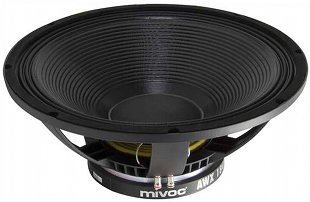open a bigger photoopen a bigger photo

recommended cabinet 1:
closed cabinet with 63 L volume
from 69/44 Hz (-3dB/-8dB)

recommended cabinet 2:
223 L volume bass reflex cabinet
with 4x HP100 reflex tube, 22 cm long.
from 35/28 Hz (-3dB/-8dB).

Mivoc AWX 184 MK II

order no. not-available- not available

44 cm bass driver with high quality cone. The basket is made of die-cast.

• power handling (continuous/programme) = 600/1200 W
• frequency range = 28-500 Hz
• resonance frequency fs = 32 Hz
• impedance R = 4 Ohm
• sound pressure level SPL = 98 dB (2,83V; 1m)
• DC resistance Re = 3,5 Ohm
• force factor BL = 18,5 N/A
• voice coil inductance L = 0,6 mH
• effective piston radiating area Sd = 1232 cm2
• effective mechanical mass incl. air load mms = 188 g
• equivalent volume of compliance Vas = 280 l
• total Q factor Qts = 0,36 (Qms=6,1, Qes=0,38)
• voice coil diameter = 100 mm
• maximum peak linear excursion vibration xlin = +/- 5,5 mm
• mounting diameter d = 420 mm
• overall diameter d = 459 mm
• mounting depth (not countersunk) t = 193 mm
PDF data sheet

Mivoc XAW 320 PR

order no. not-available- not available